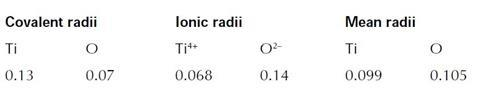One coat or two?

Calculate the thickness of a paint film and estimate roughly its thickness in titanium atoms/ions.

## Introduction

Teachers who have not used the problems before should read the section Using the problems before starting.

## Prior knowledge

Familiarity with the concept of ionic and covalent radii. A detailed knowledge is unnecessary as students are encouraged to consult textbooks and data books during the exercise.

## Resources

Students will have to find the covering power of paint by looking at a tin or a maker’s colour card. Some suitable charts or tins should be available. Scientific calculators and tables giving both atomic and ionic radii are required.

## Possible solutions

(i) The recommended covering power was 16 m2 per litre (dm3) (103 m3) of paint for one particular brand of gloss paint.

Thickness = 103/16

= 6 x 105 m

= 6 x 103 cm or 6 x 102 mm.

This assumes that there is no loss of thickness on drying.

(ii) Students may look up the rutile structure. This is relatively unusual with 6 : 3 coordination (six oxygen ions round each titanium ion, and three titanium ions around each oxygen ion). However, using this information for this calculation would be like using an analytical balance to weigh out a kilogram of potatoes – it is unnecessarily precise for our purposes!

The sizes of titanium and oxygen can be used (figures in nm):Since the bonding will be polar covalent, it is reasonable to average the figures for each element hence the radii are approximately the same, 0.1 nm. Thus the atom centres are 0.2 nm apart. As there are twice as many oxygens as titaniums, one third of the thickness could be regarded as titanium, ie

6 x 105

number of titaniums = ———— x 1/3

0.2 x 109

= 105 or 100 000

This figure assumes the paint contains only rutile; assuming that 50 % is rutile gives approximately 50 000 titanium atoms as the answer to the question.

## Suggested approach

During trialling the following instructions were given to students and proved to be extremely effective:

1. Working as a group, discuss the problem and try the calculation. You can divide the work amongst you if you wish, but keep one another informed of progress.

Such discussion can play a vital part in working out possible solutions to problems like this one. Several minds working on a problem together can stimulate the ideas that one on its own could not manage. About 10 minutes should be spent on this initially with further discussion as required.

Remember that exact answers are not always appropriate – you may have to use your judgement to make sensible estimates.

1. Write a brief account of what you did. You should explain how you decided on the estimates you had to make.
2. Working as a group, prepare a short (ca 5-minute maximum) presentation to give to the rest of the class. If possible, all group members should take part: any method of presentation (such as a blackboard, overhead projector, etc) can be used.

Outline the problem and your solutions and explain the approximations you had to make. After the presentation, be prepared to accept and answer questions and to discuss what you did with the rest of the class.